#### You may also like### Prime Magic

Place the numbers 1, 2, 3,..., 9 one on each square of a 3 by 3 grid so that all the rows and columns add up to a prime number. How many different solutions can you find?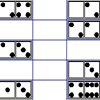### Domino Magic Rectangle

An ordinary set of dominoes can be laid out as a 7 by 4 magic rectangle in which all the spots in all the columns add to 24, while those in the rows add to 42. Try it! Now try the magic square...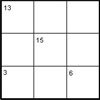### Intersection Sums Sudoku

A Sudoku with clues given as sums of entries.

# Difference Sudoku

##### Age 14 to 16 Challenge Level:

If you can manage to reduce the possibilities of one cell to 2 or 3 numbers, you could try solving the puzzle with each of those values in turn. If  you reach a contradiction (for example a number is repeated in a row, column or square) then you can rule out that value and try one of the others instead.

Here's an example of a way to start this puzzle. Let's look at this section of the grid: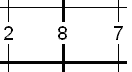We need two numbers that differ by 8, they can only be 1 and 9.
But which way round  should they go?
Let's try both possibilities, writing one pair at the top of the cells and the other at the bottom: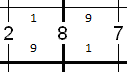Then we can use the '7' to determine the value of the next cell for each of our possibilities: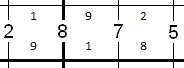We can keep going in this way with two possible solutions emerging until one of them ends in a contradiction.

Rosie and Zoe solved this Sudoku. They kept a record of the order in which they filled the Sudoku and wrote it in the boxes. Their journey can be found here. This is just one possible route through the problem.